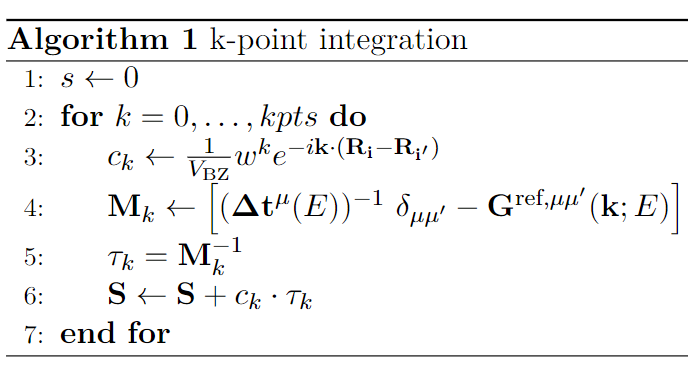# JuKKR kloop (openmp)

Version's name: JuKKR kloop (openmp) ; a version of the juKKR kloop program.
Implemented best practices: Upper level parallelisation ·

This version of the JuKKR-kloop kernel represents the original implementation of the k-point integration loop as found in the KKRhost code of the JuKKR code family. For simplicity it performs the k-point integration only for two selected energy points. These energy points are taken from a real simulation run of KKRhost on a 3D unit cell of a crystal lattice containing 4 gold atoms.

Algorithmically, the k-point integration loop looks as follows:The variables in the algorithm have the following types: | Type | Variable | | :——: | :—-: | | Scalar | $$c_k$$, $$V_{BZ}$$, $$w^k$$, $$e$$ | | Vector | $$\textbf{k}$$, $$\textbf{R}_i$$, $$\textbf{R}_{i'}$$ | | Matrix | $$\textbf{M}_k$$, $$\textbf{\Delta t}_k$$, $$\delta_{\mu \mu'}$$, $$\textbf{G}_k$$, $$\textbf{\tau}_k$$, $$\textbf{S}_k$$ |

First the exponential prefactor $$c_k$$ is constructed. The computation of the matrix $M_k$ involves an inverse Fourier transformation of $$G^{ref}$$ to get $$G^{ref, \mu\mu'}$$. Afterwards the inversion of $$M_k$$ is done by computing an LU decomposition using the LAPACK routine $$\texttt{zgetrf}$$.

In this version of the kernel the outer loop over all k-points is parallelized using OpenMP worksharing !\$omp parallel for. So the k-points are now partitioned among OpenMP threads as well. For the LU decompositions in line 5 the serial version of the Intel MKL is used now.

This implementation has the following benefits in terms of performance:
On the one hand False-Sharing effects when computing the LU decomposition of the matrices $$M_k$$ are avoided as only a single OpenMP thread accesses a single $$M_k$$ now. As a result a higher number of instructions per cycle (IPC) is yielded. Also the number of IPC scales perfectly with an increasing number of OpenMP threads now which was not the case in the original implementation (master version).
On the other hand the degree of parallelism of the whole k-point integration code has been increased. In the original implementation computation of the coefficients $$c_k$$ as well as computing the matrices $$M_k$$ were only performed by the master thread in serial. Now these computations are also done in parallel. As a result the serial fraction of the code is significantly reduced. By applying Amdahl’s law this means that the code can scale much better now with an increasing number of OpenMP threads.

The following experiments have been registered: